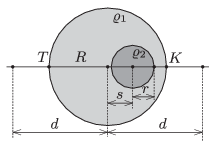Mathematical and Physical Journal
for High Schools
Issued by the MATFUND Foundation
 Already signed up? New to KöMaL?

#Problem P. 4676. (November 2014)

P. 4676. The density of a uniform sphere of radius $\displaystyle R$ is $\displaystyle \varrho_1$, except for a smaller spherical region at which the density is $\displaystyle \varrho_2$ The distance between the centres of the two spheres is $\displaystyle s$.$\displaystyle a)$ What is the acceleration due to gravity along the line which joins the centres of the spheres at a point which is at a distance of $\displaystyle d\ge R$ from the centre of the greater sphere?

$\displaystyle b)$ Let $\displaystyle s=R/2$ and $\displaystyle r=R/4$ be. In this case the acceleration due to gravity at point $\displaystyle K$ is 10% greater then at point $\displaystyle T$ shown in the figure. What is the ratio of $\displaystyle \varrho_2/\varrho_1$?

(4 pont)

Deadline expired on December 10, 2014.

### Statistics:

 48 students sent a solution. 4 points: Ardai István Tamás, Asztalos Bogdán, Balogh Menyhért, Bartók Imre, Berta Dénes, Blum Balázs, Bugár 123 Dávid, Csenger Géza, Csire Roland, Di Giovanni Márk, Fehér 169 Szilveszter, Fehér Balázs, Fekete Panna, Forrai Botond, Holczer András, Jakus Balázs István, Kormányos Hanna Rebeka, Lőrincz Zoltán, Marosvári Kristóf, Molnár 157 Marcell, Olosz Balázs, Orosz Bálint, Pázmán Előd, Sal Kristóf, Szentivánszki Soma , Szépfalvi Bálint, Tomcsányi Gergely, Wiandt Péter. 3 points: Bencsik Bálint, Gróf Tamás, Juhász 326 Dániel, Kaposvári Péter, Körmöczi Dávid, Molnár Elizabet, Németh Flóra Boróka. 2 points: 7 students. 1 point: 6 students.

Problems in Physics of KöMaL, November 2014